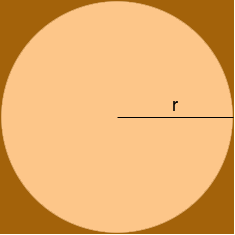SEARCH HOMEMath Central Quandaries & QueriesQuestion from mae, a student: what are the formulas on getting the area of the square outside an inscribed circular region?Hi Mae,

The area of a rectangle is the length times the width. In a square the length and width are equal so the area of a square is the length times itself.

Suppose the radius of the circle is r.What is the length of a side of the square?

PennyMath Central is supported by the University of Regina and The Pacific Institute for the Mathematical Sciences.